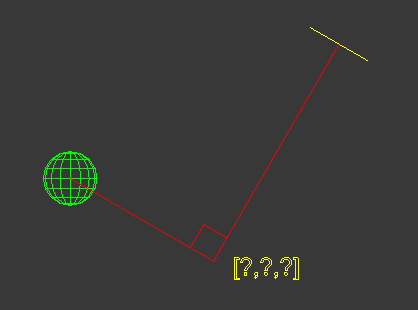# Finding the right angle

I've im given a plane [yellow object in image] and a sphere [green object], how can i find the position of the right angle between the two objects? as demonstrated in the image below. I know 3 things which i assume will help find the solution

1. The position of the plane
2. The Normal of the plane
3. The position of the sphere```/* Test Scene Setup */
delete objects
pln = plane width:5 length:5 pos:[0,10,10] wirecolor:yellow
rotate pln (angleaxis 30 [1,0,0])
s1 = sphere pos:[0,30,0] wirecolor:red radius:2

center = pln.pos
normal = pln.transform.row3```
AttachmentSize
findangle.png3.42 KB

## Comment viewing options### Completed!

nothing a little trig couldn't do

```fn GetVectorsAngle v1 v2 =
(
theAngle = acos(dot (normalize v1) (normalize v2))
)

fn PlaceAlongVector pos:[0,0,0] vec:[0,0,1] offset:0 =
(
pos + (normalize (vec)) * offset
)

/* Test Scene Setup */
clearlistener()
format "\n"
delete objects
pln = plane width:5 length:5 pos:[0,10,10] wirecolor:yellow
rotate pln (angleaxis 30 [1,0,0])
s1 = sphere pos:[0,30,0] wirecolor:red radius:2

center = pln.pos
normal = pln.transform.row3

/* Find the length to create a right traingle */
-- get angle difference between 2 vectors
difVector = s1.pos - pln.pos-- vector between plane and ball
objVector = pln.transform.row3 -- vector using normal of plane
dist = distance pln.pos s1.pos
calcAng = GetVectorsAngle difVector objVector

-- calculate the length of side to create a right angle
len = cos(calcAng) * dist
-- place point which would create a rightAngle at the sphere
cornerPos = PlaceAlongVector pos:pln.pos vec:objVector offset:len
point pos:cornerPos size:2 wirecolor:yellow```

John Martini
Digital Artist
http://www.JokerMartini.com (new site)### that is an interesting

that is an interesting approach but id like to avoid doing the intersect ray stuff

John Martini
Digital Artist
http://www.JokerMartini.com (new site)### .### aside from what im trying to

aside from what im trying to do on CGTalk, I'm still curious to know how one would find the right angle 'pos' like demonstrated in the picture.

John Martini
Digital Artist
http://www.JokerMartini.com (new site)### `

this is a way around but its very simple:
-it just copy your plane to center of sphere
-enlarge plane
-find intersection between normal or (-normal) vector and new plane

```/* Test Scene Setup */
clearlistener()
delete objects
pln = plane width:5 length:5 pos:[0,10,10] wirecolor:yellow
rotate pln (angleaxis 30 [1,0,0])
s1 = sphere pos:[0,30,0] wirecolor:red radius:2

center = pln.pos
normal = pln.transform.row3

pln2 = copy pln
pln2.pos = s1.pos
pln2.width = 999999
pln2.length = 999999

if (a = intersectRay pln2 (ray center normal)) == undefined do a = intersectRay pln2 (ray center -normal)
-- if intersection is not found because normal direction is other way
-- it checks for intersection with flipped normal

pointPos = a.pos

delete pln2

a = point pos:pointPos```### Thanks for the alternate

Thanks for the alternate solution bud. I figured it out if you look at my first post.

John Martini
Digital Artist
http://www.JokerMartini.com (new site)